# The Lehman Sculpture and the Isotropic Vector Matrix

by Kirby Urner
First posted: June 25, 2000
Last modified: June 25, 2000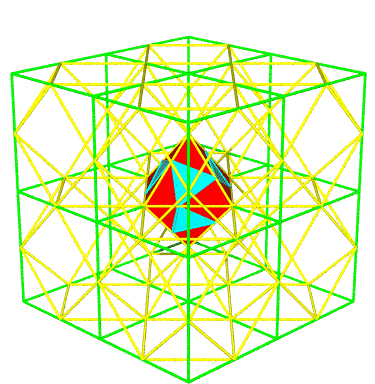Focus shifted to the vertex where 8 2F cubes meet. This could be the locus of a new penta-matrix -- with 2F cube corners at the centers of those currently displayed.The spheres shown belong to an IVM with more spheres at all the 2F cube mid-edges (the vertices of the cuboctahedra).

IVM = 2F cube centers + 2F cube mid-edges.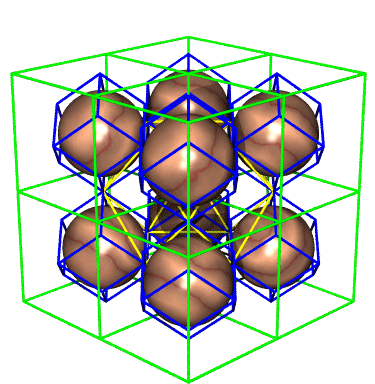2F cubes with nuclear spheres inscribed in rhombic dodecahedra (volume 6).

The centered yellow cuboctahedron indicates where spheres of an alternate IVM would be: at cube mid-faces, as well as wherever 8 2F cubes meet.

Alternate IVM = 2F cube corners + 2F cube mid-faces.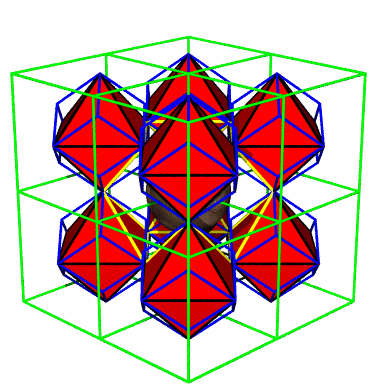The red octahedra (volume 4) appear as the long diagonals of rhombic dodecahedra.

The alternate IVM with a sphere at the center of the yellow cuboctahedron would have additional spheres at the ends of all of these long diagonals (i.e 2F cube mid-faces).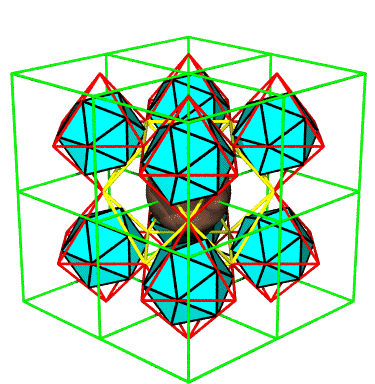Same as above but with the octahedra in wireframe, and with inscribed icosahedra.

For further reading:

Collaborative Projects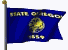A Mathematical Canvas